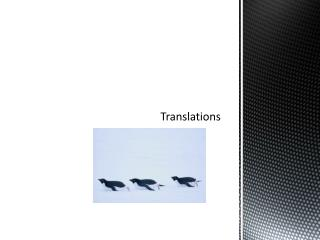Download PresentationTranslationsTranslations - PowerPoint PPT Presentation

Download PresentationTranslations
An Image/Link below is provided (as is) to download presentation

Download Policy: Content on the Website is provided to you AS IS for your information and personal use and may not be sold / licensed / shared on other websites without getting consent from its author. While downloading, if for some reason you are not able to download a presentation, the publisher may have deleted the file from their server.

- - - - - - - - - - - - - - - - - - - - - - - - - - - E N D - - - - - - - - - - - - - - - - - - - - - - - - - - -
Presentation Transcript

1. Translations

2. A translation is the transformation that maps every two points P and Q in the plane to point P’ and Q’ so that the following are true:

3. A translation is an isometry! An isometry preserves…. 1. 2. 3. 4.

4. The vertices of ∆KLM are K (3,3) , L (5,4) , and M (0,6). Find the new vertices after each slide. Verbally Algebraically Left two units ( x, y) ( ____, _____ ) K’ ( _____, ______ ) L’ ( _____ , _____ ) M’ ( ______ , ______ ) Down five units ( x, y) ( ____, _____ ) K’ ( _____, ______ ) L’ ( _____ , _____ ) M’ ( ______ , ______)

5. The coordinates of the endpoints of are P ( 1,2 ) and Q (4,6 ). M is the midpoint of . Find the coordinates of M ( _____ , _____). PQ = ________ ( x, y ) ⇢ ( x – 3 , y +1 ) P’ ⇢ ( _______, ________) ; Q’ ( _____, ______) M’ ⇢ ( ________, _________) d. P’Q’ = __________ M’Q’ = __________

6. Let’s extend this topic…. If lines k and m are parallel, then a reflection in the line k followed by a reflection in line m is a ___________________. is ________________ to k and m PP’ = ______, where d is the distance between k and m.

7. How many congruent segments can you find? Does DE = CF? What is the length of ? What do you notice about PQTW?

8. A third way is to describe a translation is by using a vector. • As we know , we can describe translations verbally… • 2 units up , 4 units down • We can describe translations algebraically…. • ( x , y ) ⇢ ( x + 2 , y – 4 )

9. A vector is a quantity that has both direction and magnitude, or size. It is represented by an arrow drawn between 2 points. How could we describe direction and magnitude?

10. The diagram shows a vector. The initial point, or starting point, of the vector is P. The terminal point, or ending point, is Q. The vector is named .

11. The horizontal component of is 7. The vertical component of is 4. The component form of a vector combines the horizontal and vertical components. Component form of is . ( 7 , 4 ) is an ordered pair ……. is the component form of a vector.

12. The component form of is . Use to translate ∆ABC. A ( ______, _______ ) B ( ______, _______ ) C ( ______, _______ ) A’ ( ______, _______ ) B’ ( ______, _______ ) C’ ( ______, _______ )

13. A boat travels a straight path between two islands, A and D. When the boat is 3 miles east and 2 miles north of its starting point it encounters a storm at point B. The storm pushes the boat off course to point C. ( 8 , 4.5 ) D . Draw 2 vectors that represent the problem stated. Write the component form for the 2 vectors drawn. ( 3 , 2 ) C B . • . ( 4 , 2 ) A . ( 0 , 0 )

14. The final destination is 8 miles east and 4.5 miles north of the starting point. Draw the vector needed to get the boat to the island. Write the component form of the vector that describes the path the boat can follow to arrive at its destination. ( 8 , 4.5 ) D . ( 3 , 2 ) C B . • . ( 4 , 2 ) A . ( 0 , 0 )

15. How far did the did the boat travel? How much farther did the boat travel due to the storm? ( 8 , 4.5 ) D . ( 3 , 2 ) C B . • . ( 4 , 2 ) A . ( 0 , 0 )

16. Guided practice – Lesson 7.4 A Assignment – Lesson 7.4 B